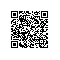# 详解Graph Embedding经典方法：算法原理、代码实现与应用样例# 导读

Graph Embedding 技术将图中的节点以低维稠密向量的形式进行表达，要求在原始图中相似 ( 不同的方法对相似的定义不同 ) 的节点其在低维表达空间也接近。得到的表达向量可以用来进行下游任务，如节点分类，链接预测，可视化或重构原始图等。

# DeepWalk

DeepWalk 的思想类似 word2vec，使用图中节点与节点的共现关系来学习节点的向量表示。那么关键的问题就是如何来描述节点与节点的共现关系，DeepWalk 给出的方法是使用随机游走 (RandomWalk) 的方式在图中进行节点采样。

RandomWalk 是一种可重复访问已访问节点的深度优先遍历算法。给定当前访问起始节点，从其邻居中随机采样节点作为下一个访问节点，重复此过程，直到访问序列长度满足预设条件。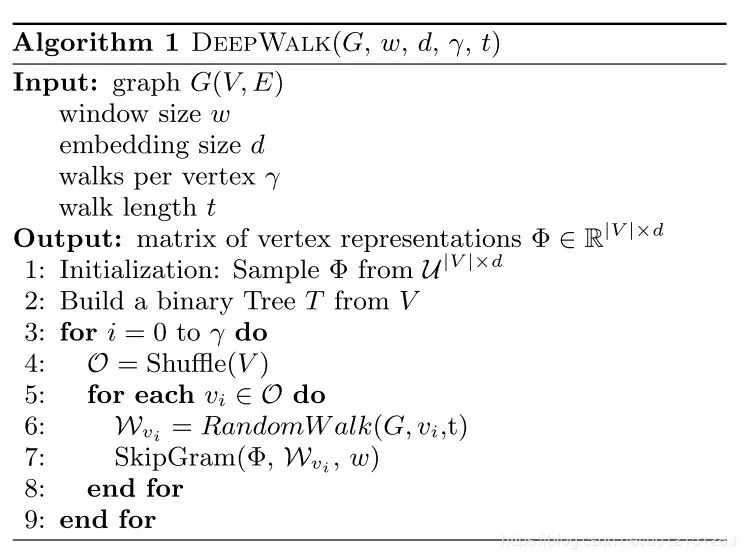▐ DeepWalk 核心代码

DeepWalk 算法主要包括两个步骤，第一步为随机游走采样节点序列，第二步为使用 skip-gram modelword2vec 学习表达向量。

• 构建同构网络，从网络中的每个节点开始分别进行 Random Walk 采样，得到局部相关联的训练数据
• 对采样数据进行 SkipGram 训练，将离散的网络节点表示成向量化，最大化节点共现，使用 Hierarchical Softmax 来做超大规模分类的分类器

▐ Random Walk

deepwalk_walk方法对应上一节伪代码中第6行，_simulate_walks对应伪代码中第3行开始的外层循环。最后的Parallel为多进程并行时的任务分配操作。

def deepwalk_walk(self, walk_length, start_node):

walk = [start_node]

while len(walk) < walk_length:
cur = walk[-1]
cur_nbrs = list(self.G.neighbors(cur))
if len(cur_nbrs) > 0:
walk.append(random.choice(cur_nbrs))
else:
break
return walk

def _simulate_walks(self, nodes, num_walks, walk_length,):
walks = []
for _ in range(num_walks):
random.shuffle(nodes)
for v in nodes:
walks.append(self.deepwalk_walk(alk_length=walk_length, start_node=v))
return walks

results = Parallel(n_jobs=workers, verbose=verbose, )(
delayed(self._simulate_walks)(nodes, num, walk_length) for num in
partition_num(num_walks, workers))

walks = list(itertools.chain(*results))

▐ Word2vec

from gensim.models import Word2Vec
w2v_model = Word2Vec(walks,sg=1,hs=1)

▐ DeepWalk 应用

https://github.com/shenweichen/GraphEmbedding

G = nx.read_edgelist('../data/wiki/Wiki_edgelist.txt',create_using=nx.DiGraph(),nodetype=None,data=[('weight',int)])

model = DeepWalk(G,walk_length=10,num_walks=80,workers=1)
model.train(window_size=5,iter=3)
embeddings = model.get_embeddings()

evaluate_embeddings(embeddings)
plot_embeddings(embeddings)

▐ 分类任务结果

micro-F1 : 0.6674
macro-F1 : 0.5768

▐ 可视化结果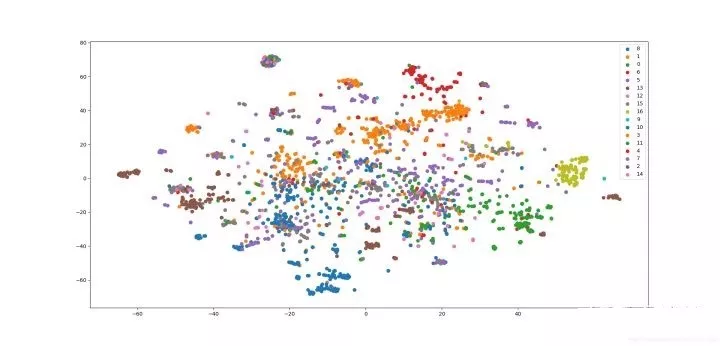# LINE

LINE也是一种基于邻域相似假设的方法，只不过与DeepWalk使用DFS构造邻域不同的是，LINE可以看作是一种使用BFS构造邻域的算法。此外，LINE还可以应用在带权图中(DeepWalk仅能用于无权图)。

▐ LINE 算法原理

1. 一种新的相似度定义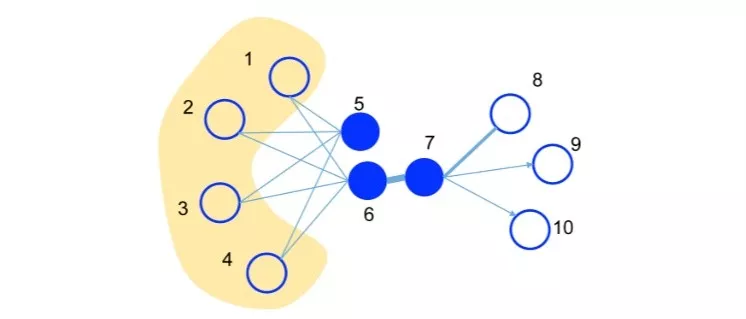✎ first-order proximity

1阶相似度用于描述图中成对顶点之间的局部相似度，形式化描述为若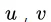之间存在直连边，则边权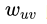即为两个顶点的相似度，若不存在直连边，则1阶相似度为0。如上图，6和7之间存在直连边，且边权较大，则认为两者相似且1阶相似度较高，而5和6之间不存在直连边，则两者间1阶相似度为0。

✎ second-order proximity

2. 优化目标

✎ 1st-order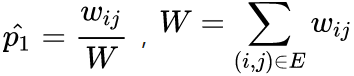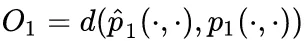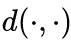是两个分布的距离，常用的衡量两个概率分布差异的指标为KL散度，使用KL散度并忽略常数项后有：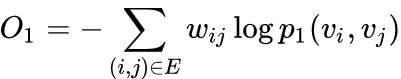1st order 相似度只能用于无向图当中。

✎ 2nd-order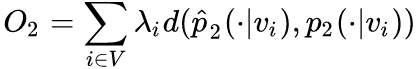，其中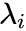为控制节点重要性的因子，可以通过顶点的度数或者PageRank等方法估计得到。

**3. 优化技巧
**

✎ Negative sampling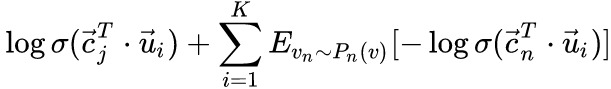，k是负边的个数。

✎ Edge Sampling

Alias Method:时间复杂度O(1)的离散采样方法

https://zhuanlan.zhihu.com/p/54867139

4. 其他问题

✎ 低度数顶点

✎ 新加入顶点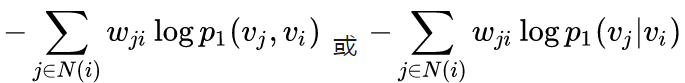▐ LINE核心代码

1. 模型和损失函数定义

LINE使用梯度下降的方法进行优化，直接使用tensorflow进行实现，就可以不用人工写参数更新的逻辑了~

def line_loss(y_true, y_pred):
return -K.mean(K.log(K.sigmoid(y_true*y_pred)))def create_model(numNodes, embedding_size, order='second'):

v_i = Input(shape=(1,))
v_j = Input(shape=(1,))

first_emb = Embedding(numNodes, embedding_size, name='first_emb')
second_emb = Embedding(numNodes, embedding_size, name='second_emb')
context_emb = Embedding(numNodes, embedding_size, name='context_emb')

v_i_emb = first_emb(v_i)
v_j_emb = first_emb(v_j)

v_i_emb_second = second_emb(v_i)
v_j_context_emb = context_emb(v_j)

first = Lambda(lambda x: tf.reduce_sum(
x*x, axis=-1, keep_dims=False), name='first_order')([v_i_emb, v_j_emb])
second = Lambda(lambda x: tf.reduce_sum(
x*x, axis=-1, keep_dims=False), name='second_order')([v_i_emb_second, v_j_context_emb])

if order == 'first':
output_list = [first]
elif order == 'second':
output_list = [second]
else:
output_list = [first, second]

model = Model(inputs=[v_i, v_j], outputs=output_list)

2. 顶点负采样和边采样

def _gen_sampling_table(self):

# create sampling table for vertex
power = 0.75
numNodes = self.node_size
node_degree = np.zeros(numNodes)  # out degree
node2idx = self.node2idx

for edge in self.graph.edges():
node_degree[node2idx[edge]
] += self.graph[edge][edge].get('weight', 1.0)

total_sum = sum([math.pow(node_degree[i], power)
for i in range(numNodes)])
norm_prob = [float(math.pow(node_degree[j], power)) /
total_sum for j in range(numNodes)]

self.node_accept, self.node_alias = create_alias_table(norm_prob)

# create sampling table for edge
numEdges = self.graph.number_of_edges()
total_sum = sum([self.graph[edge][edge].get('weight', 1.0)
for edge in self.graph.edges()])
norm_prob = [self.graph[edge][edge].get('weight', 1.0) *
numEdges / total_sum for edge in self.graph.edges()]

self.edge_accept, self.edge_alias = create_alias_table(norm_prob)

▐ LINE 应用

https://github.com/shenweichen/GraphEmbedding

G = nx.read_edgelist('../data/wiki/Wiki_edgelist.txt',create_using=nx.DiGraph(),nodetype=None,data=[('weight',int)])

model = LINE(G,embedding_size=128,order='second')
model.train(batch_size=1024,epochs=50,verbose=2)
embeddings = model.get_embeddings()

evaluate_embeddings(embeddings)
plot_embeddings(embeddings)

▐ 分类任务结果

micro-F1: 0.6403
macro-F1:0.5286

▐ 可视化结果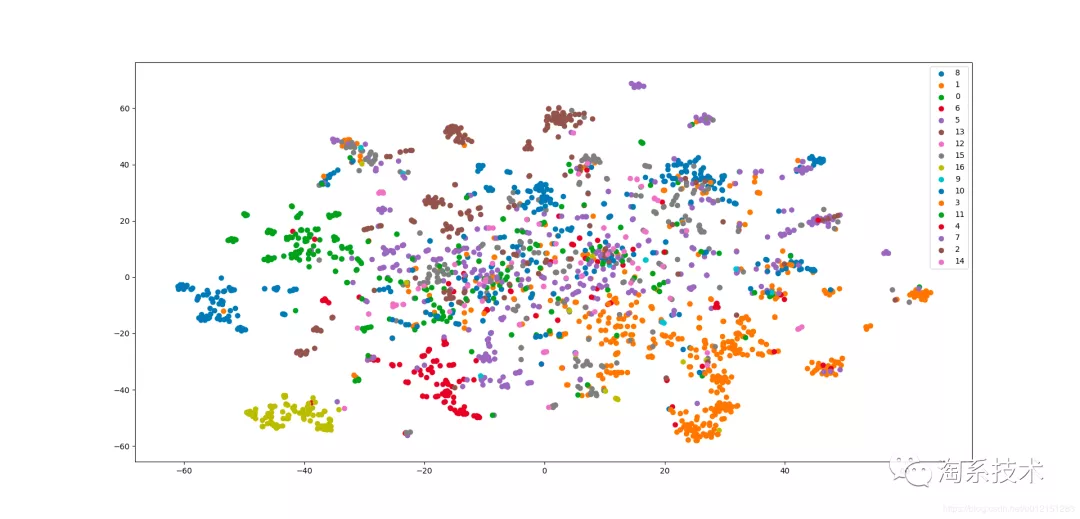# node2vec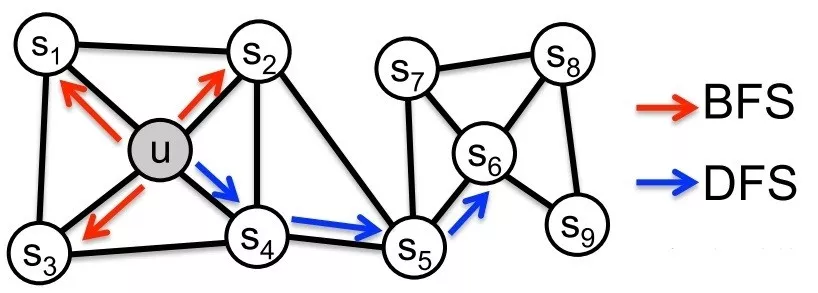node2vec是一种综合考虑DFS邻域和BFS邻域的graph embedding方法。简单来说，可以看作是deepwalk的一种扩展，是结合了DFS和BFS随机游走的deepwalk。

▐ node2vec 算法原理

1. 优化目标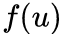是将顶点u映射为embedding向量的映射函数,对于图中每个顶点u，定义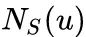为通过采样策略s采样出的顶点u的近邻顶点集合。

node2vec优化的目标是给定每个顶点条件下，令其近邻顶点（如何定义近邻顶点很重要）出现的概率最大。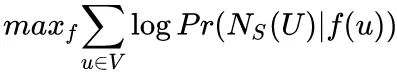• 条件独立性假设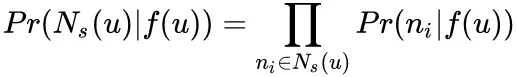• 特征空间对称性假设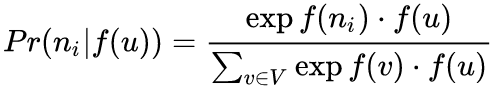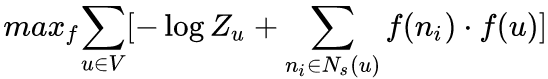1. 顶点序列采样策略

node2vec依然采用随机游走的方式获取顶点的近邻序列，不同的是node2vec采用的是一种有偏的随机游走。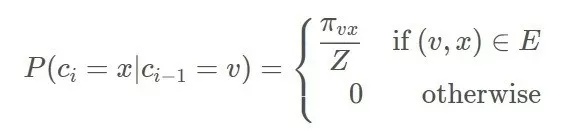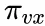是顶点 v和顶点x之间的未归一化转移概率， z是归一化常数。

node2vec引入两个超参数 和来控制随机游走的策略，假设当前随机游走经过边（t，v）到达顶点v设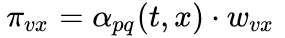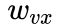是顶点v和x之间的边权。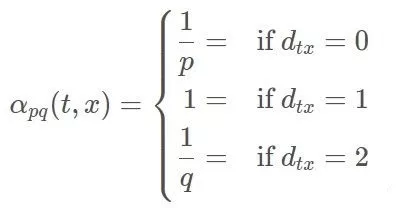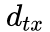为顶点t和顶点x之间的最短路径距离。

• Return parameter,p

• In-out papameter,q

q控制着游走是向外还是向内，若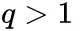，随机游走倾向于访问和t接近的顶点(偏向BFS)。若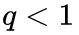，倾向于访问远离t的顶点(偏向DFS)。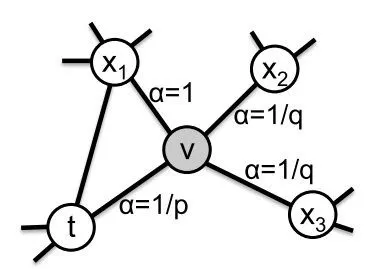3. 学习算法

Alias Method:时间复杂度O(1)的离散采样方法
https://zhuanlan.zhihu.com/p/54867139

▐ node2vec 核心代码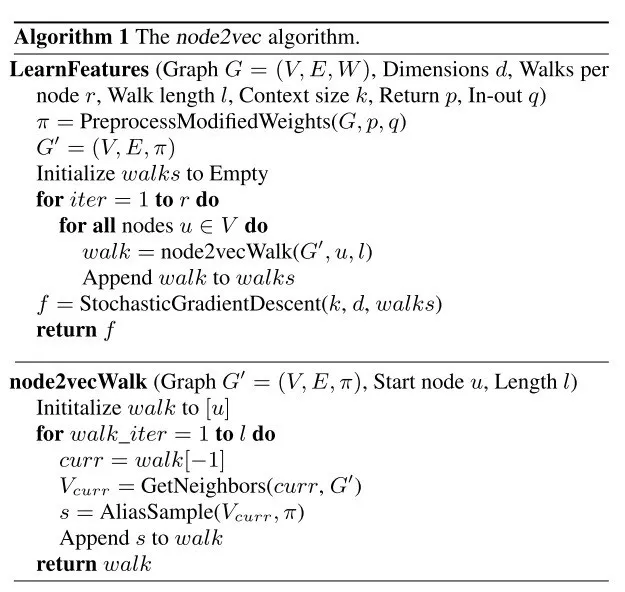1. node2vecWalk

def node2vec_walk(self, walk_length, start_node):
G = self.G
alias_nodes = self.alias_nodes
alias_edges = self.alias_edges
walk = [start_node]
while len(walk) < walk_length:
cur = walk[-1]
cur_nbrs = list(G.neighbors(cur))
if len(cur_nbrs) > 0:
if len(walk) == 1:
walk.append(cur_nbrs[alias_sample(alias_nodes[cur], alias_nodes[cur])])
else:
prev = walk[-2]
edge = (prev, cur)
next_node = cur_nbrs[alias_sample(alias_edges[edge],alias_edges[edge])]
walk.append(next_node)
else:
break
return walk

2. 构造采样表
preprocess_transition_probs分别生成alias_nodes和alias_edges，alias_nodes存储着在每个顶点时决定下一次访问其邻接点时需要的alias表（不考虑当前顶点之前访问的顶点）。alias_edges存储着在前一个访问顶点为t，当前顶点为 时决定下一次访问哪个邻接点时需要的alias表。

get_alias_edge方法返回的是在上一次访问顶点 t，当前访问顶点x为时到下一个顶点的未归一化转移概率: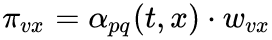def get_alias_edge(self, t, v):
G = self.G
p = self.p
q = self.q
unnormalized_probs = []
for x in G.neighbors(v):
weight = G[v][x].get('weight', 1.0)# w_vx
if x == t:# d_tx == 0
unnormalized_probs.append(weight/p)
elif G.has_edge(x, t):# d_tx == 1
unnormalized_probs.append(weight)
else:# d_tx == 2
unnormalized_probs.append(weight/q)
norm_const = sum(unnormalized_probs)
normalized_probs = [float(u_prob)/norm_const for u_prob in unnormalized_probs]
return create_alias_table(normalized_probs)def preprocess_transition_probs(self):
G = self.G
alias_nodes = {}
for node in G.nodes():
unnormalized_probs = [G[node][nbr].get('weight', 1.0) for nbr in G.neighbors(node)]
norm_const = sum(unnormalized_probs)
normalized_probs = [float(u_prob)/norm_const for u_prob in unnormalized_probs]
alias_nodes[node] = create_alias_table(normalized_probs)
alias_edges = {}
for edge in G.edges():
alias_edges[edge] = self.get_alias_edge(edge, edge)
self.alias_nodes = alias_nodes
self.alias_edges = alias_edges
return

▐ node2vec 应用

https://github.com/shenweichen/GraphEmbedding

G = nx.read_edgelist('../data/wiki/Wiki_edgelist.txt',create_using=nx.DiGraph(),nodetype=None,data=[('weight',int)])

model = Node2Vec(G,walk_length=10,num_walks=80,p=0.25,q=4,workers=1)
model.train(window_size=5,iter=3)
embeddings = model.get_embeddings()

evaluate_embeddings(embeddings)
plot_embeddings(embeddings)

▐ 分类任务

micro-F1: 0.6757 macro-F1: 0.5917

▐ 可视化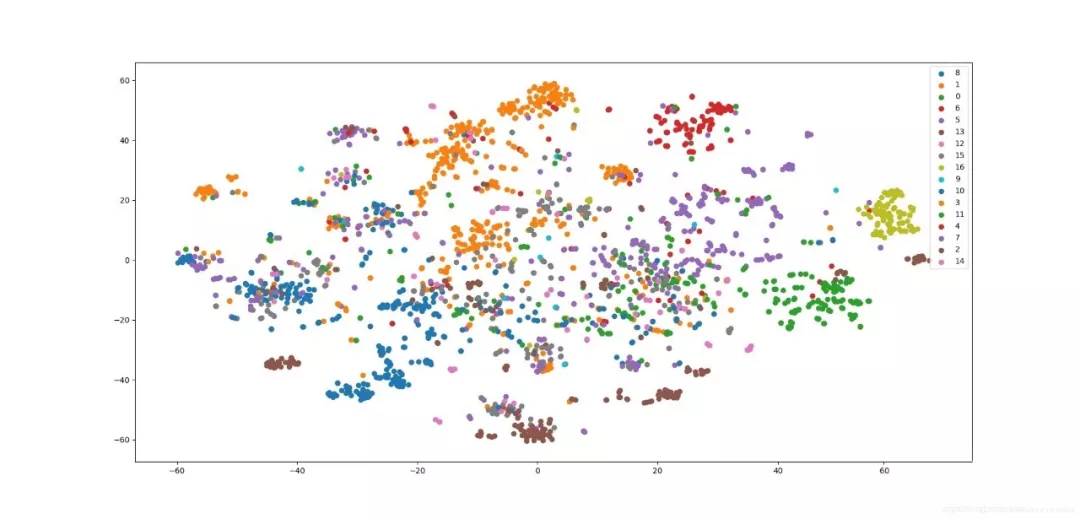# SDNE

SDNE（Structural Deep Network Embedding ）是和node2vec并列的工作，均发表在2016年的KDD会议中。可以看作是基于LINE的扩展，同时也是第一个将深度学习应用于网络表示学习中的方法。

SDNE使用一个自动编码器结构来同时优化1阶和2阶相似度(LINE是分别优化的)，学习得到的向量表示能够保留局部和全局结构，并且对稀疏网络具有鲁棒性。

▐ SDNE 算法原理

SDNE中的相似度定义和LINE是一样的。简单来说，1阶相似度衡量的是相邻的两个顶点对之间相似性。2阶相似度衡量的是，两个顶点他们的邻居集合的相似程度。

2阶相似度优化目标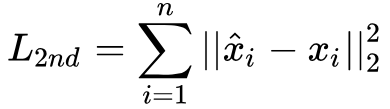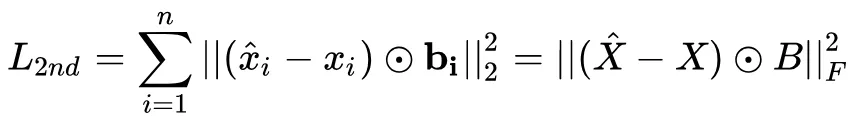1阶相似度优化目标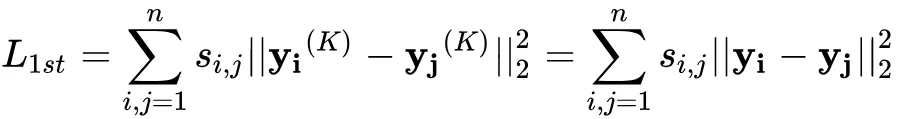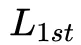还可以表示为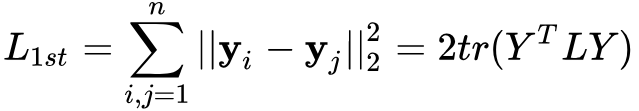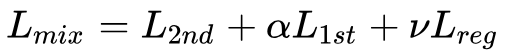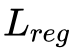是正则化项，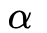为控制1阶损失的参数，v为控制正则化项的参数。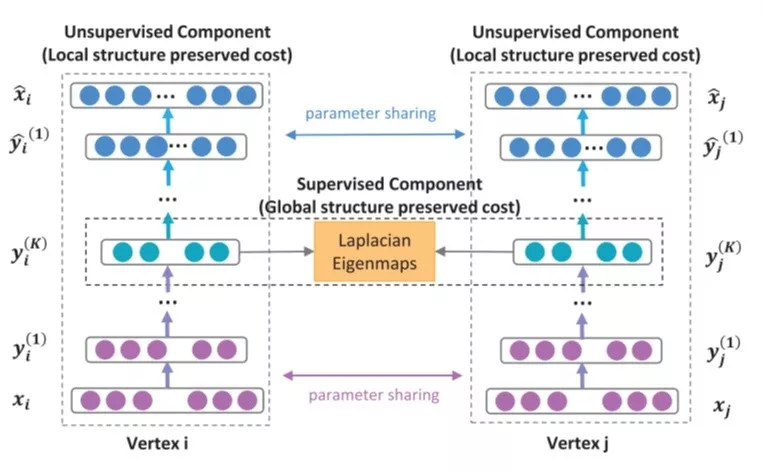▐ 实现

l_2nd是2阶相似度对应的损失函数，参数beta控制着非零元素的惩罚项系数。y_true和y_pred分别是输入的邻接矩阵和网络重构出的邻接矩阵。

l_1st是1阶相似度对应的损失函数，参数alpha控制着其在整体损失函数中的占比。

def l_2nd(beta):
def loss_2nd(y_true, y_pred):
b_ = np.ones_like(y_true)
b_[y_true != 0] = beta
x = K.square((y_true - y_pred) * b_)
t = K.sum(x, axis=-1, )
return K.mean(t)
return loss_2nd

def l_1st(alpha):
def loss_1st(y_true, y_pred):
L = y_true
Y = y_pred
batch_size = tf.to_float(K.shape(L))
return alpha * 2 * tf.linalg.trace(tf.matmul(tf.matmul(Y, L, transpose_a=True), Y)) / batch_size
return loss_1st

create_model函数创建SDNE模型，l1和l2分别为模型的正则化项系数，模型的输入A为邻接矩阵，L为拉普拉斯矩阵。输出A_为重构后的邻接矩阵，Y为顶点的embedding向量。

def create_model(node_size, hidden_size=[256, 128], l1=1e-5, l2=1e-4):
A = Input(shape=(node_size,))
L = Input(shape=(None,))
fc = A
for i in range(len(hidden_size)):
if i == len(hidden_size) - 1:
fc = Dense(hidden_size[i], activation='relu',kernel_regularizer=l1_l2(l1, l2),name='1st')(fc)
else:
fc = Dense(hidden_size[i], activation='relu',kernel_regularizer=l1_l2(l1, l2))(fc)
Y = fc
for i in reversed(range(len(hidden_size) - 1)):
fc = Dense(hidden_size[i], activation='relu',kernel_regularizer=l1_l2(l1, l2))(fc)
A_ = Dense(node_size, 'relu', name='2nd')(fc)
model = Model(inputs=[A, L], outputs=[A_, Y])
return model

▐ 应用

https://github.com/shenweichen/GraphEmbedding

▐ 分类任务

micro-F1: 0.6341 macro-F1: 0.4962

▐ 可视化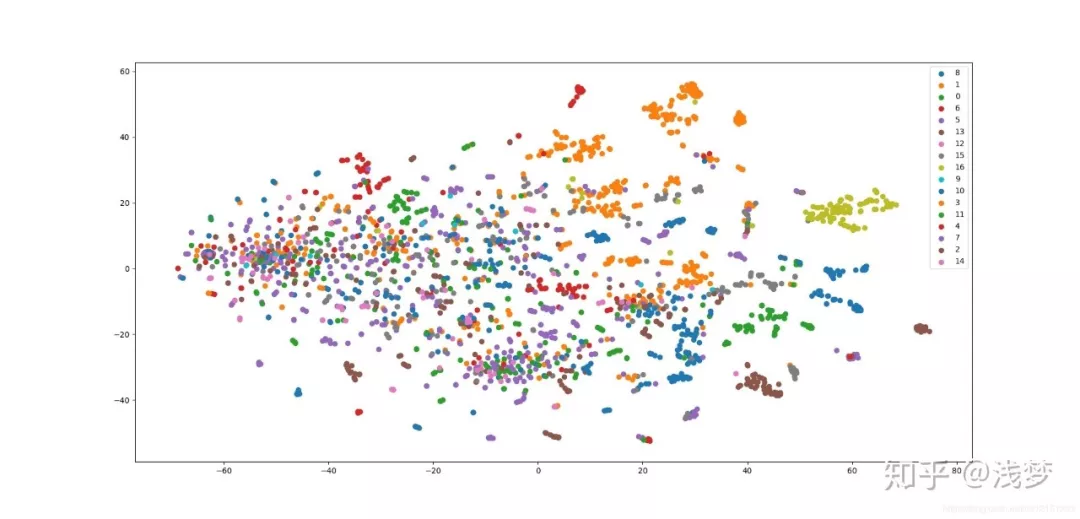# Struc2Vec

▐ Struc2Vec算法原理

Struc2Vec是从空间结构相似性的角度定义顶点相似度的。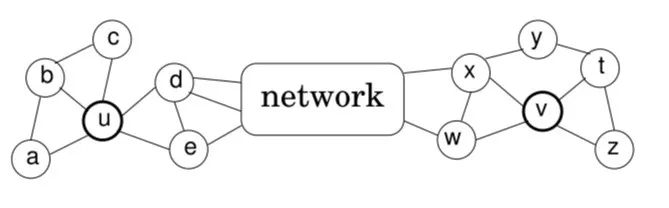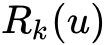表示到顶点u距离为k的顶点集合，则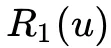表示是u的直接相连近邻集合。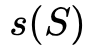表示顶点集合S的有序度序列。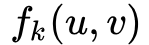表示顶点u和v之间距离为k（这里的距离k实际上是指距离小于等于k的节点集合）的环路上的结构距离(注意是距离，不是相似度)。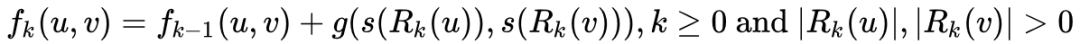.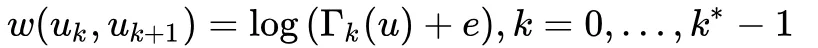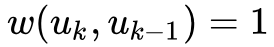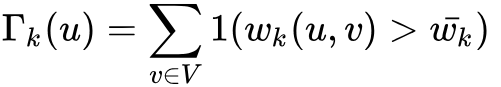，就是第k层所有边权的平均值。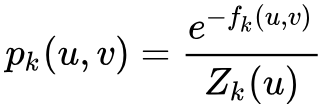其中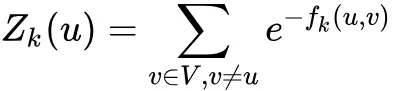是第k层中关于顶点u的归一化因子。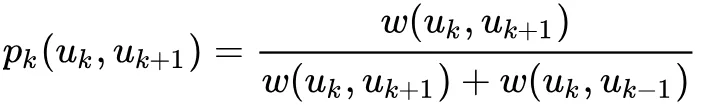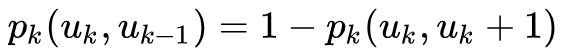• OPT1 有序度序列长度优化
前面提到过对于每个顶点在每一层都有一个有序度序列，而每一个度序列的空间复杂度为O(n)。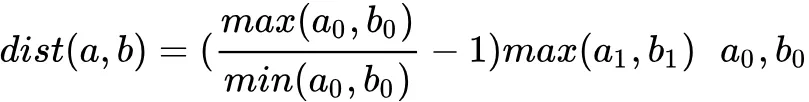为度数， a1，b1为度的出现次数。

• OPT2 相似度计算优化

• OPT3 限制层次带权图层数
层次带权图M中的层数是由图的直径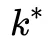决定的。但是对很多图来说，图的直径会远远大于顶点之间的平均距离。

▐ Struc2Vec 核心代码

Struc2Vec的实现相比于前面的几个算法稍微复杂一些，这里我主要说下大体思路，对一些细节有疑问的同学可以邮件或者私信我~

1. 获取每一层的顶点对距离
2. 根据顶点对距离构建带权层次图
3. 在带权层次图中随机游走采样顶点序列

def _get_order_degreelist_node(self, root, max_num_layers=None):
if max_num_layers is None:
max_num_layers = float('inf')

ordered_degree_sequence_dict = {}
visited = [False] * len(self.graph.nodes())
queue = deque()
level = 0
queue.append(root)
visited[root] = True

while (len(queue) > 0 and level <= max_num_layers):

count = len(queue)
if self.opt1_reduce_len:
degree_list = {}
else:
degree_list = []
while (count > 0):

top = queue.popleft()
node = self.idx2node[top]
degree = len(self.graph[node])

if self.opt1_reduce_len:
degree_list[degree] = degree_list.get(degree, 0) + 1
else:
degree_list.append(degree)

for nei in self.graph[node]:
nei_idx = self.node2idx[nei]
if not visited[nei_idx]:
visited[nei_idx] = True
queue.append(nei_idx)
count -= 1
if self.opt1_reduce_len:
orderd_degree_list = [(degree, freq)
for degree, freq in degree_list.items()]
orderd_degree_list.sort(key=lambda x: x)
else:
orderd_degree_list = sorted(degree_list)
ordered_degree_sequence_dict[level] = orderd_degree_list
level += 1

return ordered_degree_sequence_dict

def _compute_ordered_degreelist(self, max_num_layers):

degreeList = {}
vertices = self.idx # self.g.nodes()
for v in vertices:
degreeList[v] = self._get_order_degreelist_node(v, max_num_layers)
return degreeList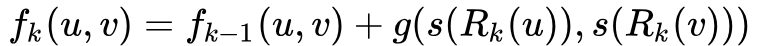def compute_dtw_dist(part_list, degreeList, dist_func):
dtw_dist = {}
for v1, nbs in part_list:
lists_v1 = degreeList[v1] # lists_v1 :orderd degree list of v1
for v2 in nbs:
lists_v2 = degreeList[v2] # lists_v1 :orderd degree list of v2
max_layer = min(len(lists_v1), len(lists_v2)) # valid layer
dtw_dist[v1, v2] = {}
for layer in range(0, max_layer):
dist, path = fastdtw(
lists_v1[layer], lists_v2[layer], radius=1, dist=dist_func)
dtw_dist[v1, v2][layer] = dist
return dtw_dist

def _compute_structural_distance(self, max_num_layers, workers=1, verbose=0,):

if os.path.exists(self.temp_path+'structural_dist.pkl'):
structural_dist = pd.read_pickle(
self.temp_path+'structural_dist.pkl')
else:
if self.opt1_reduce_len:
dist_func = cost_max
else:
dist_func = cost

if os.path.exists(self.temp_path + 'degreelist.pkl'):
degreeList = pd.read_pickle(self.temp_path + 'degreelist.pkl')
else:
degreeList = self._compute_ordered_degreelist(max_num_layers)
pd.to_pickle(degreeList, self.temp_path + 'degreelist.pkl')

if self.opt2_reduce_sim_calc:
degrees = self._create_vectors()
degreeListsSelected = {}
vertices = {}
n_nodes = len(self.idx)
for v in self.idx: # c:list of vertex
nbs = get_vertices(
v, len(self.graph[self.idx2node[v]]), degrees, n_nodes)
vertices[v] = nbs # store nbs
degreeListsSelected[v] = degreeList[v] # store dist
for n in nbs:
# store dist of nbs
degreeListsSelected[n] = degreeList[n]
else:
vertices = {}
for v in degreeList:
vertices[v] = [vd for vd in degreeList.keys() if vd > v]

results = Parallel(n_jobs=workers, verbose=verbose,)(
delayed(compute_dtw_dist)(part_list, degreeList, dist_func) for part_list in partition_dict(vertices, workers))
dtw_dist = dict(ChainMap(*results))

structural_dist = convert_dtw_struc_dist(dtw_dist)
pd.to_pickle(structural_dist, self.temp_path +
'structural_dist.pkl')

return structural_dist

layers_adj存储着每一层中每个顶点的邻接点，layers_distances存储着每一层每个顶点对的结构化距离。_get_transition_probs只做了一件事情，就是逐层的计算顶点之间的边权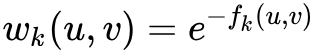，并生成后续采样需要的alias表。

def _get_transition_probs(self, layers_adj, layers_distances):
layers_alias = {}
layers_accept = {}

for layer in layers_adj:

neighbors = layers_adj[layer]
layer_distances = layers_distances[layer]
node_alias_dict = {}
node_accept_dict = {}
norm_weights = {}

for v, neighbors in neighbors.items():
e_list = []
sum_w = 0.0

for n in neighbors:
if (v, n) in layer_distances:
wd = layer_distances[v, n]
else:
wd = layer_distances[n, v]
w = np.exp(-float(wd))
e_list.append(w)
sum_w += w

e_list = [x / sum_w for x in e_list]
norm_weights[v] = e_list
accept, alias = create_alias_table(e_list)
node_alias_dict[v] = alias
node_accept_dict[v] = accept

pd.to_pickle(
norm_weights, self.temp_path + 'norm_weights_distance-layer-' + str(layer)+'.pkl')

layers_alias[layer] = node_alias_dict
layers_accept[layer] = node_accept_dict

return layers_accept, layers_alias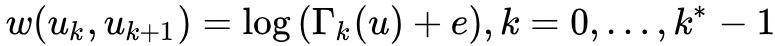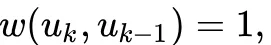def prepare_biased_walk(self,):

sum_weights = {}
sum_edges = {}
average_weight = {}
gamma = {}
layer = 0
while (os.path.exists(self.temp_path+'norm_weights_distance-layer-' + str(layer))):
probs = pd.read_pickle(
self.temp_path+'norm_weights_distance-layer-' + str(layer))
for v, list_weights in probs.items():
sum_weights.setdefault(layer, 0)
sum_edges.setdefault(layer, 0)
sum_weights[layer] += sum(list_weights)
sum_edges[layer] += len(list_weights)

average_weight[layer] = sum_weights[layer] / sum_edges[layer]

gamma.setdefault(layer, {})

for v, list_weights in probs.items():
num_neighbours = 0
for w in list_weights:
if (w > average_weight[layer]):
num_neighbours += 1
gamma[layer][v] = num_neighbours

layer += 1

pd.to_pickle(average_weight, self.temp_path + 'average_weight')
pd.to_pickle(gamma, self.temp_path + 'gamma.pkl')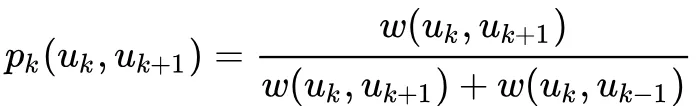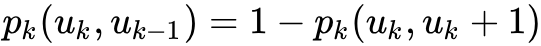def _exec_random_walk(self, graphs, layers_accept,layers_alias, v, walk_length, gamma, stay_prob=0.3):
initialLayer = 0
layer = initialLayer

path = []
path.append(self.idx2node[v])

while len(path) < walk_length:
r = random.random()
if(r < stay_prob): # same layer
v = chooseNeighbor(v, graphs, layers_alias,
layers_accept, layer)
path.append(self.idx2node[v])
else: # different layer
r = random.random()
try:
x = math.log(gamma[layer][v] + math.e)
p_moveup = (x / (x + 1))
except:
print(layer, v)
raise ValueError()

if(r > p_moveup):
if(layer > initialLayer):
layer = layer - 1
else:
if((layer + 1) in graphs and v in graphs[layer + 1]):
layer = layer + 1

return path

▐ Struc2Vec 应用

Struc2Vec应用于无权无向图(带权图的权重不会用到，有向图会当成无向图处理)，主要关注的是图中顶点的空间结构相似性，这里我们采用论文中使用的一个数据集。该数据集是一个机场流量的数据集，顶点表示机场，边表示两个机场之间存在航班。机场会被打上活跃等级的标签。

https://github.com/shenweichen/GraphEmbedding

▐ 分类

Struc2Vec结果 micro-F1: 0.7143, macro-F1: 0.7357

Node2Vec结果 micro-F1: 0.3571, macro-F1: 0.3445

▐ 可视化

Struc2Vec结果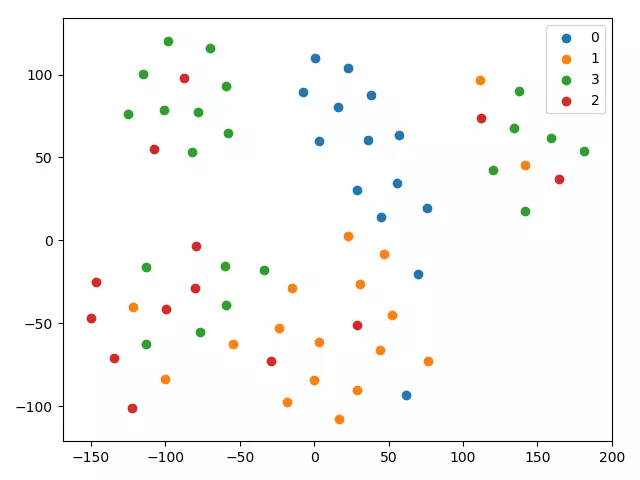Node2Vec结果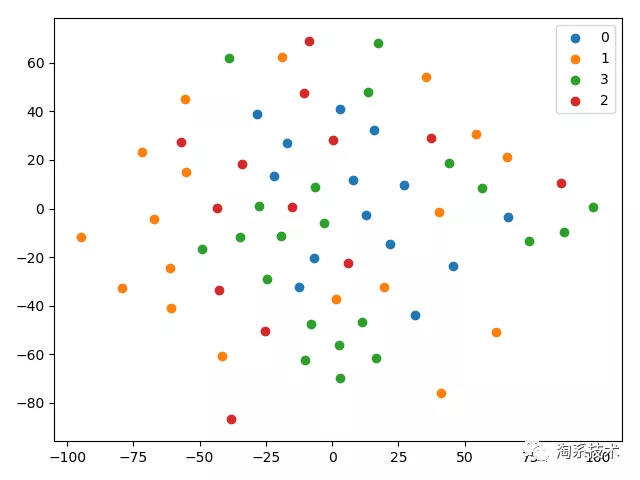1. [Perozzi B, Al-Rfou R, Skiena S. Deepwalk: Online learning of social representations[C]//Proceedings of the 20th ACM SIGKDD international conference on Knowledge discovery and data mining. ACM, 2014: 701-710.](链接地址http://www.perozzi.net/publications/14_kdd_deepwalk.pdf)
2. Graph Neural Network Review
3. Tang J, Qu M, Wang M, et al. Line: Large-scale information network embedding[C]//Proceedings of the 24th International Conference on World Wide Web. International World Wide Web Conferences Steering Committee, 2015: 1067-1077.
4. [Grover A, Leskovec J. node2vec: Scalable Feature Learning for Networks[C]// Acm Sigkdd International Conference on Knowledge Discovery & Data Mining. 2016.](链接地址https://www.kdd.org/kdd2016/papers/files/rfp0218-groverA.pdf)
5. [Wang D, Cui P, Zhu W. Structural deep network embedding[C]//Proceedings of the 22nd ACM SIGKDD international conference on Knowledge discovery and data mining. ACM, 2016: 1225-1234.](链接地址https://www.kdd.org/kdd2016/papers/files/rfp0191-wangAemb.pdf)
6. struc2vec: Learning Node Representations from Structural Identity